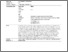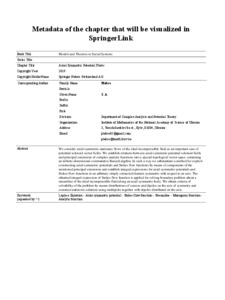# Metadata of the chapter that will be visualized in SpringerLink

Плакса, С. А. (2018) Metadata of the chapter that will be visualized in SpringerLink. Models and Theories in Social Systems, 1. pp. 1-32.Preview
Text
Download (674kB) | Preview

## Abstract

We consider axial-symmetric stationary flows of the ideal incompressible fluid as an important case of potential solenoid vector fields. We establish relations between axial-symmetric potential solenoid fields and principal extensions of complex analytic functions into a special topological vector space containing an infinite-dimensional commutative Banach algebra. In such a way we substantiate a method for explicit constructing axial-symmetric potentials and Stokes flow functions by means of components of the mentioned principal extensions and establish integral expressions for axial-symmetric potentials and Stokes flow functions in an arbitrary simply connected domain symmetric with respect to an axis. The obtained integral expression of Stokes flow function is applied for solving boundary problem about a streamline of the ideal incompressible fluid along an axial-symmetric body. We obtain criteria of solvability of the problem by means distributions of sources and dipoles on the axis of symmetry and construct unknown solutions using multipoles together with dipoles distributed on the axis.

Item Type: Article Laplace Equation - Axial-symmetric potential - Stokes flow function - Streamline - Monogenic function - Analytic function Q Science > QA Mathematics > Mathematical Analysis Faculty of Physics and Mathematics > Department of Mathematical Analysis Ірина Ігорівна Таргонська 26 Feb 2019 08:59 26 Feb 2019 09:38 http://eprints.zu.edu.ua/id/eprint/28407

### Actions (login required)View Item

### Downloads

Downloads per month over past year

View more statistics